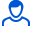Content: teorver_5560.doc (29.00 KB)

Positive responses: 0
Negative responses: 0

Refunds: 05560. x̅=112.2, n=27, σ=5. Given the standard deviation σ=5, the sample mean x̅в=112.2 and the sample size n=27 of a normally distributed feature of the general population. Find confidence intervals for estimating the general mean x̅g with a given reliability γ=0.95.
Detailed solution. Decorated in Microsoft Word 2003 (Quest decided to use the formula editor)
No feedback yet